## Section 1. Introduction to finite mixture models and mixComp

Mixture models have been used extensively whenever data is assumed to have been sampled from a population consisting of several homogeneous subpopulations, called components within this framework. Depending on the application, the amount of a priori information on the mixture may vary substantially. Above all, one differentiates between parametric and nonparametric models. This distinction separates models where the family of distributions to which the components belong is known to be some parametric family (or where the practitioner wants to suppose this to be the case) from those where no such parametric assumptions are imposed. A second distinction can be made to distinguish models with a known number of components from those where this information has to be estimated from the data. To refer to this number, the terms order, complexity and number of components will be used interchangeably. If the number of components in a finite mixture model is known, an EM algorithm can be used to find the MLE of all unknown parameters. However, in many applications there is no possibility of knowing the complexity a priori and the objective becomes to estimate the number of components or to find the most parsimonious mixture (i.e. the one with the fewest possible components) which still provides a satisfactory fit to the data. These are the kind of tasks the mixComp package was developed to deal with in the realm of parametric finite mixtures.

In what follows we write simply density keeping in mind that it may be taken with respect either to the Lebesgue or the counting measure. Stated formally, a distribution $$F$$ is called a finite mixture if its density is of the form $f(x) = \sum_{i=1}^p w_i g_i(x),$ where $$p \in \mathbb{N}$$ is the mixture complexity, $$(w_1, \dots w_p : \sum_{i=1}^p w_i = 1, w_i \geq 0,$$ for $$i=1,\dots,p)$$ are the component weights and the density $$g_i(x)$$ is the $i$th component of the mixture. As the scope of mixComp is limited to parametric mixtures, we replace $$g_i(x)$$ by a parametric density $$g(x; \theta_i)$$ indexed by the (possibly multivariate, say $$d$$-dimensional) parameter $$\theta_i$$ in the parameter space $$\Theta \subseteq \mathbb{R}^d$$.

Given some complexity $$j$$, the two relevant parameter spaces can therefore be defined as $\Theta_j = \{\theta_1 \dots \theta_j: \theta_i \in \Theta \subseteq \mathbb{R}^d, \text{ for } i = 1,\dots,j\}$ and $W_j = \{w_1, \dots, w_j: \sum_{i=1}^j w_i = 1, w_i \geq 0, \text{ for } i = 1,\dots,j\}.$

Throughout this document, it is assumed that the family of the component densities $$\{g(x; \theta):\theta \in \Theta\}$$ is known, but the component parameters $$\mathbf{\theta} = (\theta_1, \dots, \theta_p) \in \Theta_p$$, the component weights $$\textbf{w} = (w_1, \dots, w_p) \in W_p$$ and the mixture complexity $$p \in \mathbb{N}$$ are unknown, with $$p$$ being the parameter of interest. Assume now that $$F$$ is a finite mixture distribution with density $f(x) = \sum_{i=1}^p w_i g(x; \theta_i)$ and $$\textbf{X} = \{X_1, \dots, X_n\}$$ is an i.i.d. sample of size $$n$$ from $$F$$. The mixComp package aims to estimate the smallest such $$p$$ on the basis of $$\textbf{X}$$, either on its own or by simultaneously estimating the weights $$w_i$$ and the component parameters $$\theta_i$$, $$i \in 1, \dots, p$$.

In this setup, it seems natural to test for the number of components by comparing two consecutive models (with $$j$$ and $$j+1$$ components, say) and regarding the mixture with $$j$$ components as the imposition of a null hypothesis on the larger model (intuitively, the null hypothesis $H_0: \left\{ \exists i \in \{1, 2, \dots, j+1\}: w_{i} = 0 \right\}$ comes to mind). Traditionally, the problem of testing between nested models may be approached by applying the generalized likelihood ratio test and referring to a table of the $$\chi^2_r$$ distribution to assess significance, where $$r$$ is given by the number of constraints imposed on $$H_1$$ in order to arrive at $$H_0$$. However, in the context of mixture models, there is no unique way of obtaining $$H_0$$ from $$H_1$$. As an example, the two null hypotheses $$H_0: w_{j+1} = 0$$ and $$H_0: \theta_{j+1} = \theta_{1}$$ both yield the smaller model, demonstrating the difficulties of applying this method. This problem has been studied extensively in the literature and numerous other approaches to complexity estimation have been suggested, laying the theoretical foundation for the algorithms described further.

This document discusses various categories of functions found in the mixComp package, ranging from methods based on Hankel matrices, to techniques based upon distances between densities and likelihood ratio tests. The examples provided in the first sections all contain mixtures of “standard” distributions for which evaluation of the density, cumulative distribution function and quantile function as well as random variate generation may be performed using the functions from the stats package. The last section illustrates how the mixComp package can be used to estimate the complexity of any mixture as long as the user provides functions generating random variates from the component distribution and valuating the density thereof.

Two main features distinguish this package from other mixture-related R [@R] packages: Firstly, it is focused on the estimation of the complexity rather than the component weights and parameters. While these are often estimated as a by-product, all methods contained in mixComp are based on theory specifically developed to consistently estimate the number of components in the mixture of interest. Secondly, it is applicable to parametric mixtures well beyond those whose component distributions are included in the stats package, making it more customizable than most packages for model-based clustering.

The packages mixtools [see @mixtools] and flexmix [see @flexmix1; @flexmix2; @flexmix3] should both be mentioned at this point: aside from mixtools's focus on mixture-of-regressions and non-parametric mixtures which are less relevant to this package, it is widely used to fit (multivariate) normal, multinomial or gamma mixtures with the EM algorithm. Notably, it also contains routines for selecting the number of components based on information criteria and parametric bootstrapping of the likelihood ratio test statistic values. However, they are limited to multinomial and (a variety of) normal mixtures as well as mixtures-of-regressions. Second, while flexmix was developed to deal with mixtures-of-regression, it sets itself apart from other packages by its extensibility, a design principle that we also aimed for when creating the mixComp package. Other widely used packages dealing with mixture models are mclust [@mclust], which fits mixtures of Gaussians using the EM algorithm, and mixdist [@mixdist], which is used for grouped conditional data. Interested readers can find a comprehensive list of mixture-related packages on the CRAN Task View: Cluster Analysis and Finite Mixture Models website.

## Section 2. Objects and functions defined in mixComp

Table 1 depicts five object classes defined in mixComp. The first two respectively represent a finite mixture distribution and a random sample drawn from such a distribution. The Mix object is printed as a matrix of component weights and parameters and is plotted as the density of the specified mixture, showing the overall as well as the component densities. The rMix object prints as the vector of observations and plots as a histogram, showcasing the individual components as well as the full sample. Both objects contain a number of attributes giving additional information, details of which can be found in the corresponding R help files.

#### Table 1: Objects and functions defined in mixComp

Object class Created via Description
Mix Mix Represents a finite mixture
rMix rMix Randomly generated data from a finite mixture
datMix datMix or RtoDat Observed data from (presumably) a finite mixture
hankDet nonparamHankel Vector of estimated Hankel matrix determinants
paramEst paramHankel(.scaled), L2(.boot).disc, hellinger(.boot).disc, hellinger(.boot).cont or mix.lrt Complexity estimate $$\hat{p}$$, together with estimates of the weights $$\hat{\mathbf{w}}$$ and the component parameters $$\hat{\mathbf{\theta}}$$

The generation of an object of class Mix hinges on three central arguments: a string dist specifying the name of the family of component densities (or kernels) $$\{g(x;\theta):\theta \in \Theta \}$$, a vector w giving the weights $$w_i$$ and a list theta.list (the component parameters can also be supplied via the ... argument) containing the parameters of the component densities $$\theta_i, i \in 1, \dots, p$$. While the creation of Mix objects is mostly straightforward, two things should be noted in this regard: First, the mixComp procedures will search for functions called rdist and ddist in the accessible namespaces. For most “standard” distributions, these functions are contained in the stats package and do not need to be user-written (compare with the Section 6). To make use of these functions, it is essential that the string dist is named correctly (e.g. to create a gaussian mixture on the basis of the stats package, dist has to be specified as norm instead of normal, gaussian etc. for the package to find the functions dnorm and rnorm). Second, the names of the list elements of theta.list(for the names of the ... arguments) have to match the names of the formal arguments of the functions ddist and rdist exactly (e.g. for a gaussian mixture, the list elements have to be named mean and sd, as these are the formal arguments used by rnorm and dnorm).

The following example creates two Mix objects, a $$3$$-component mixture of normal distributions and a $$3$$-component mixture of Poisson distributions.

library(mixComp)

set.seed(0)
normLocMix <- Mix("norm", w = c(0.3, 0.4, 0.3), mean = c(10, 13, 17), sd = c(1, 1, 1))
poisMix <- Mix("pois", w = c(0.45, 0.45, 0.1), lambda = c(1, 5, 10))


The created Mix objects can then be plotted.

plot(normLocMix, main = "Three component normal mixture")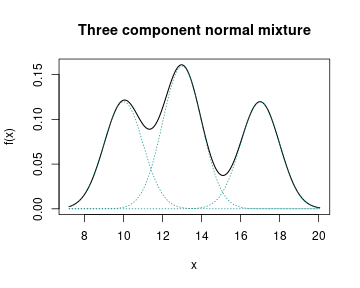plot(poisMix, main = "Three component poisson mixture")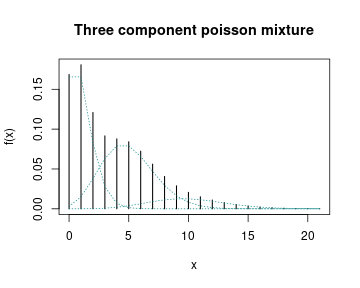If required, random samples can be generated from these mixtures.

# generate random samples:
normLocRMix <- rMix(1000, obj = normLocMix)
poisRMix <- rMix(1000, obj = poisMix)


The histograms of the generated random samples can be plotted.

# plot the histograms of the random samples:
plot(normLocRMix, main = "Three component normal mixture")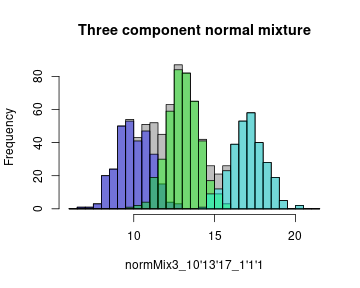plot(poisRMix, main = "Three component poisson mixture")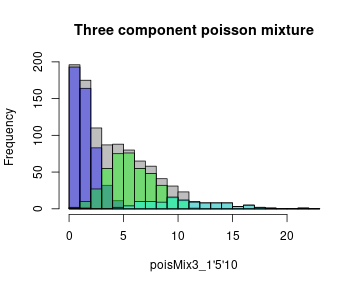The third object class shown in Table 1, called datMix, represents the data vector $$\textbf{X}$$ based on which the mixture complexity is supposed to be estimated. These objects are most central to the package, as every procedure estimating the order of a mixture takes a datMix object as input. Apart from $$\mathbf{X}$$, it contains other “static” information needed for the estimation procedure (in contrast to “tuning parameters”, which can be changed with every function call. An example of such a tuning parameter is the number of bootstrap replicates for a function employing a bootstrap procedure). A brief overview of which “static” attributes need to be supplied for each complexity estimation routine is given in Table 2.

#### Table 2: Inputs needed for different functions contained in mixComp

R function dist theta.bound.list MLE.function Hankel.method Hankel.function
nonparamHankel x x x
nonparamHankel(.scaled) x x o + i (optional) x x
L2(.boot).disc x x i (optional)
hellinger(.boot).disc x x i (optional)
hellinger(.boot).cont x x i (optional)
mix.lrt x x o + i (optional)

In the table, “o” and “i” respectively stand for input used during optimization and initialization. As a simple example of a given dataset to which mixture models have been applied extensively, take the Old Faithful dataset [@R; @faithful1; @faithful2]. In the context of mixture model estimation, the variable waiting, which gives the time in minutes between eruptions of the Old Faithful geyser in the Yellowstone National Park, is often considered to be the variable of interest. To estimate the number of components of the mixture distribution that provides a suitable approximation to the waiting data via mixComp, the raw data vector of observations has to be converted to a datMix object first. For the sake of exposition we specify all arguments of the datMix function, starting with the vector of observations $$\textbf{X}$$ and the string dist, specifying $$\{g(x;\theta):\theta \in \Theta \}$$. As has often been done in the relevant literature, we assume that the data comes from a normal mixture.

obs <- faithful$waiting norm.dist <- "norm"  Second, a named list of length $$d$$ containing the bounds of $$\theta \in \Theta \subseteq \mathbf{R}^d$$ has to be created. In this example, $$\theta = \{\mu, \sigma\} \in \Theta = \mathbf{R} \times (0, \infty) \subseteq \mathbf{R}^2$$. norm.bound.list <- vector(mode = "list", length = 2) names(norm.bound.list) <- c("mean", "sd") norm.bound.list$mean <- c(-Inf, Inf)
norm.bound.list$sd <- c(0, Inf)  Next, the argument MLE.function contains a single function if $$d = 1$$ or a list of functions of length $$d$$ otherwise, specifying the $$d$$ functions needed to estimate the MLE's of $$\theta$$ based on $$\textbf{X}$$ if $$p$$ were equal to 1 (i.e. the MLE's of the component distribution). If this argument is supplied and the datMix object is handed over to a complexity estimation procedure relying on optimizing over a likelihood function, the MLE.function attribute will be used for the single component case. In case the objective function is either not a likelihood or corresponds to a mixture with more than $$1$$ component, numerical optimization will be used based on Rsolnp's function solnp [@solnp, @Rsolnp]. The initial values (for the parameters of a $$j$$-component mixture, say) supplied to the solver are then calculated as follows: the data $$\textbf{X}$$ is clustered into $$j$$ groups by the function clara (of the cluster package by [@cluster] and the data corresponding to each group is given to MLE.function. The size of the groups is taken as initial component weights and the MLE's are taken as initial component parameter estimates. Specifying MLE.function is optional and if it is not, for example because the MLE solution does not exists in closed form, numerical optimization is used to find the relevant MLE's. Presuming a normal mixture, one specifies $$2$$ functions, namely the MLE of the mean $$\hat{\mu}_{MLE} = \frac{1}{n}\sum_{i = 1}^n X_i$$ and the MLE of the standard deviation $$\hat{\sigma}_{MLE} = \sqrt{\frac{1}{n}\sum_{i = 1}^n (X_i - \hat{\mu}_{MLE})^2}$$. MLE.norm.mean <- function(dat) mean(dat) MLE.norm.sd <- function(dat){ sqrt((length(dat) - 1) / length(dat)) * sd(dat) } MLE.norm.list <- list("MLE.norm.mean" = MLE.norm.mean, "MLE.norm.sd" = MLE.norm.sd)  The last two arguments, Hankel.method and Hankel.function, need to be supplied if the mixture complexity is to be estimated based on the Hankel matrix of the moments of the mixing distribution. The reader is referred to the Section 3 for further information on how these arguments are to be specified (in this case, the simplifying assumption of unit variance is made. This would be a poor choice for the waiting data, so $$p$$ should not be estimated with one of the methods using these arguments, namely nonparamHankel, paramHankel and paramHankel.scaled, see Table 2). method <- "translation" mom.std.norm <- function(j){ ifelse(j %% 2 == 0, prod(seq(1, j - 1, by = 2)), 0) }  Finally, all previously generated objects are combined to a datMix object. faithful.dM <- datMix(obs, dist = norm.dist, theta.bound.list = norm.bound.list, MLE.function = MLE.norm.list, Hankel.method = method, Hankel.function = mom.std.norm)  In the preceding example, the data vector $$\textbf{X}$$ was taken from an already existing dataset. As seen before, the rMix function can be used to generate a $$n$$-sized sample from a specific mixture. If this synthesized data is to be used in simulations (i.e. passed to one of the functions estimating the mixture complexity) an rMix object can be converted to a datMix object via the RtoDat function. Apart from dist, all datMix arguments have to be supplied to RtoDat likewise. Unlike the above mentioned objects whose creation precedes any type of mixture complexity estimation, objects of the bottom two classes (see Table 1) contain the results of the estimation procedures. Generally, the functions estimating the number of components differ in the types of families of component densities $$\{g(x;\theta):\theta \in \Theta \}$$ for which they allow and in whether they provide estimates of the weights $$\hat{w}_i$$ and the component parameters $$\hat{\theta}_i, i \in 1, \dots, \hat{p}$$, the latter determining the object class of the estimation result. These differences are shown in Table 3. The function nonparamHankel returns an object of class hankDet, which is a vector of determinants (scaled and/or penalized), each entry belonging to a certain complexity estimate. The link between these determinants and $$p$$ will be discussed in the Section 3. paramEst objects arise when using any other function estimating the mixture complexity, all of which additionally return estimates of the component weights and parameters. For both object classes, print and plot methods are available to summarize and visualize the estimation results. #### Table 3: Distribution restrictions and output types of different functions contained in mixComp R function Restrictions on the family of the component density Estimation of $$\mathbf{w}$$ and $$\mathbf{\theta}$$ nonparamHankel Compatible with explicit, translation or scale nonparamHankel(.scaled) Compatible with explicit, translation or scale x L2(.boot).disc Discrete distributions x hellinger(.boot).disc Discrete distributions x hellinger(.boot).cont Continuous distributions x mix.lrt x ## Section 3. Functions using Hankel matrices In 1997, [@hankel] showed that the order of a mixture $$p$$ is characterized by the smallest integer $$j$$ such that the determinant of the $$(j+1) \times (j+1)$$ Hankel matrix of the first $$2j$$ moments of the mixing distribution equals zero. Moreover, it can be shown that this determinant is zero for all $$j \geq p$$. Formally, for any vector $$\mathbf{c} = (c_0, \dots, c_{2k}) \in \mathbb{R}^{2k+1}$$ with $$c_0$$ equal to 1, the Hankel matrix of $$\mathbf{c}$$ is defined as the $$(k+1)\times(k+1)$$ matrix given by $H(\mathbf{c})_{i,j} = c_{i+j-2}, \quad \quad 1 \leq i,j \leq k+1.$ Now, let $$\textbf{c}^{2j+1} \in \mathbb{R}^{2j+1}$$ be the vector containing the first $$2j+1$$ (raw) moments of the mixing distribution. For finite mixture models, this amounts to $c^{2j+1}_k = \sum_{i=1}^p w_i \theta^k_i, \quad \text{ for } k \in \{0,\dots, 2j\} \text{ and } \theta_i \in \mathbb{R}, i \in \{1,\dots,p\}.$ Then, for all $$j \geq 1$$, $$H(\textbf{c}^{2j+1})$$ is non-negative and $p = \min\{j \geq 1 : \det H(\textbf{c}^{2j+1}) = 0\}.$ Making use of this fact, the first approach to estimating the order of a mixture that is implemented in mixComp relies on initially finding a consistent estimator of $$\textbf{c}^{2j+1}$$ based on $$\textbf{X}$$, say $$\hat{\textbf{c}}^{2j+1}$$, to then iteratively calculate the applicable Hankel matrix while increasing the assumed order $$j$$ until a sufficiently small value of $$\det H(\hat{\textbf{c}}^{2j+1})$$ is attained. However, since $$\det H(\hat{\textbf{c}}^{2j+1})$$ should be close to 0 for all $$j \geq p$$, this would lead to choosing $$\hat{p}$$ rather larger than the true value and it seems natural to introduce a penalty term. Therefore [@hankel] define the empirical penalized objective function as $J_n(j) := \lvert \det H(\hat{\textbf{c}}^{2j+1}) \rvert + A(j)l(n),$ with $$l(n)$$ being a positive function converging to $$0$$ as $$n\to\infty$$ and $$A(j)$$ being positive and strictly increasing. $\hat{p} := \arg\min_{j \in \mathbb{N}} J_n(j)$ is then a consistent estimator of $$p$$. As an extension to simply adding a penalty term to the determinant, a scaling approach was considered by [@lilian]. Let $$\hat{d}_j = \det H(\hat{\textbf{c}}^{2j+1})$$, $$d_j = \det H(\textbf{c}^{2j+1})$$ and $$j_m \geq p, j_m \in \mathbb{N}$$. Since the estimated moments $$\hat{\textbf{c}}^{2j+1}$$ are asymptotically normal, one can apply the delta method giving $\sqrt{n} \begin{pmatrix} \hat{d}_1-d_1\ \vdots\ \hat{d}_{p-1}-d_{p-1}\ \hat{d}_p-0\ \vdots\ \hat{d}_{j_m}-0 \end{pmatrix} \quad \overset{\mathcal{D}}{\longrightarrow} \quad \mathcal{N}(0_{j_m \times 1}, \Sigma_ {j_m \times j_m}).$ Instead of inspecting the vector $$(\hat{d}_1, \dots, \hat{d}_{j_m})$$, one could therefore also base the complexity analysis on a vector of scaled determinants, defined as $\hat{d}_{\text{scaled}}^{j_m} = \hat{\Sigma}^{-½} \begin{pmatrix} \hat{d}_1\ \vdots\ \hat{d}_{j_m}\ \end{pmatrix},$ where $$\hat{\Sigma}$$ can be calculated by a nonparametric bootstrap procedure. This approach was proposed to address the issue of determinants already being very small from the beginning (even for $$j = 1$$), which, in the simulations by [@lilian], made it hard to discern the “best” complexity estimate, a problem that was not reduced much by solely adding a penalty term. With this general framework in place, the computation now merely hinges on calculating $$\hat{\textbf{c}}^{2j+1}$$. The mixComp package offers three methods to do so. The method to use depends on the family of component densities $$\{g(x;\theta):\theta \in \Theta \}$$ and is linked to some function $$f_j(\textbf{X})$$ needed to estimate $$\hat{\textbf{c}}^{2j+1}$$. The calculation method and the relevant function are specified when creating the datMix object as arguments Hankel.method and Hankel.function. #### 1. Hankel.method = "explicit" This method can be applied when a closed form expression for estimates of the moments of the mixing distribution exists. Hankel.function then contains the function explicitly estimating $$\textbf{c}^{2j+1}$$. As an example, consider a mixture of geometric distributions, where it can be shown that $c^{2j+1}_j = 1 - \sum_{l = 0}^{j-1} f(l) = 1 - F(j-1),$ with $$F$$ the true cumulative distribution function. This expression is called $$c^{2j+1}_j$$ only for notational consisteny; the RHS is simply a closed-form expression for the $$j^{th}$$ moment of the mixing distribution of a geometric mixture. Hence one may take $\hat{c}^{2j+1}_j = 1 - \hat{F}(j-1)$ as an estimator, with $$\hat{F}$$ being the empirical distribution function. explicit.geom <- function(dat, j){ 1 - ecdf(dat)(j - 1) }  As a second example, consider what [@hankel, p. 283, equation (3)] called the “natural” estimator, i.e. using $\hat{c}^{2j+1}_j = f_j\left(\frac{1}{n} \sum_{i=1}^n \psi_j(X_i)\right)$ when $c^{2j+1}_j = f_j(\mathbb{E}[\psi_j(X_i)]).$ Note that the estimators of this form may also be supplied as Hankel.method = "explicit" with Hankel.function. For example, the “natural” estimator is applicable in the case of poisson mixtures. If $$Y \sim Pois(\lambda)$$, it is a well known fact that $\lambda^j = \mathbb{E}[Y(Y-1)\dots(Y-j+1)],$ which then suggests using $\hat{c}^{2j+1}_j = \frac{1}{n} \sum_{i=1}^n X_i(X_i-1)\dots(X_i-j+1)$ as an estimator. explicit.pois <- function(dat, j){ mat <- matrix(dat, nrow = length(dat), ncol = j) - matrix(0:(j-1), nrow = length(dat), ncol = j, byrow = TRUE) return(mean(apply(mat, 1, prod))) }  #### 2. Hankel.method = "translation" Example 3.1. in [@hankel, p.284] describes how $$\textbf{c}^{2j+1}$$ can be estimated if the family of component distributions $$(G_\theta)$$ is given by $$\text{d}G_\theta(x) = \text{d}G(x-\theta)$$, where $$G$$ is a known probability distribution whose moments can be given explicitly. In this case, a triangular linear system can be solved for the estimated moments of the mixing distribution $$\hat{\textbf{c}}^{2j+1}$$ using the empirical moments of the mixture distribution and the known moments of $$G$$. The former can be estimated from the data vector $$\textbf{X}$$ whereas the latter has to be supplied by the user. Thus, Hankel.function contains a function of $$j$$ returning the $$j^{th}$$ (raw) moment of $$G$$. As an example, consider a mixture of normal distributions with unknown mean and unit variance. Then $$G$$ is the standard normal distribution, and its $$j^{th}$$ moment $$m_j$$ is defined as $m_j= \begin{cases} 0 & \text{if } j \text{ uneven}\ (j-1)!! & \text{if } j \text{ even} .\end{cases}$ mom.std.norm <- function(j){ ifelse(j %% 2 == 0, prod(seq(1, j - 1, by = 2)), 0) }  #### 3. Hankel.method = "scale" Similarly, example 3.2. in [@hankel, p.285] describes how $$\textbf{c}^{2j+1}$$ can be estimated if the family of component distributions $$(G_\theta)$$ is given by $$\text{d}G_\theta(x) = \text{d}G(\frac{x}{\theta})$$, where $$G$$ is a known probability distribution whose moments can be given explicitly. Likewise, a triangular linear system can be solved for $$\hat{\textbf{c}}^{2j+1}$$, using the empirical moments of the mixture distribution and the known moments of $$G$$. Hankel.function contains a function of $$j$$ returning the $$j^{th}$$ moment of $$G$$. Note that squares have to be taken everywhere if for some integer $$j$$, $$m_j = 0$$ (compare with [@hankel, p.285]). As an example, consider a mixture of normal distributions with zero mean and unknown variance. Then $$G$$ is again the standard normal distribution, and its $$j^{th}$$ moment is defined as above. Coming back to the overall goal of complexity estimation, the function nonparamHankel returns all estimated determinant values corresponding to complexities up to j.max, so that the user can pick the lowest $$j$$ generating a sufficiently small determinant. The function allows the inclusion of a penalty term as a function of the sample size n and the currently assumed complexity j which will be added to the determinant value (by supplying pen.function), and/or scaling of the determinants (by setting scaled = TRUE). For scaling, a nonparametric bootstrap is used to calculate the covariance of the estimated determinants, with B being the size of the bootstrap sample. The inverse of the square root (i.e. the matrix $$S$$ such that $$A = SS$$, where $$A$$ is the (square) covariance matrix. The procedure uses expm's sqrtm [@expm]) of this covariance matrix is then multiplied with the estimated determinant vector to get the scaled determinant vector. As an example, consider data generated from a three component geometric mixture, with $$\textbf{w} = (0.1, 0.6, 0.3)$$ and $$\mathbf{\theta} = (0.8, 0.2, 0.4)$$. set.seed(1) geomMix <- Mix("geom", w = c(0.1, 0.6, 0.3), prob = c(0.8, 0.2, 0.4)) geomRMix <- rMix(1000, obj = geomMix) geom.dM <- RtoDat(geomRMix, Hankel.method = "explicit", Hankel.function = explicit.geom)  We define the penalty $$A(j)l(n)$$ as $$\frac{j\log(n)}{\sqrt{n}}$$ and scale the determinants. pen <- function(j, n){ (j * log(n)) / (sqrt(n)) } set.seed(1) geomdets_sca_pen <- nonparamHankel(geom.dM, j.max = 5, scaled = TRUE, B = 1000, pen.function = pen)  We can print and plot the results as suggested below. print(geomdets_sca_pen) #> #> Estimation of the scaled and penalized determinants for a 'geom' mixture model: #> Number of components Determinant #> 1 5.398355 #> 2 1.507395 #> 3 1.186997 #> 4 0.924233 #> 5 1.527491 plot(geomdets_sca_pen, main = "Three component geometric mixture")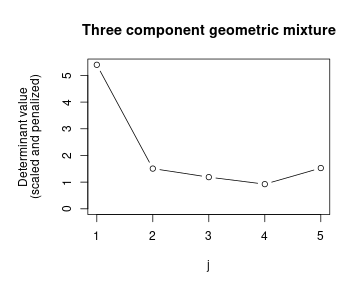As the preceding example shows, it can be quite difficult to determine the order estimate from the vector of estimated determinants alone. Thus, the package includes another option of estimating $$p$$ based on Hankel matrices, however, using a more “parametric” approach which goes hand in hand with estimating $$\mathbf{w}$$ and $$\mathbf{\theta}$$. The paramHankel procedure initially assumes the mixture to only contain a single component, setting $$j = 1$$, and then sequentially tests $$p = j$$ versus $$p = j+1$$ for $$j = 1,2, \dots$$, until the algorithm terminates. To do so, it determines the MLE for a $$j$$-component mixture $$(\hat{\mathbf{w}}^j, \hat{\mathbf{\theta}}^j) = (\hat{w}_1, \dots, \hat{w}_j, \hat{\theta}_1, \dots, \hat{\theta}_j) \in W_j \times \Theta_j$$, generates B parametric bootstrap samples of size $$n$$ from the distribution corresponding to $$(\hat{\mathbf{w}}^j, \hat{\mathbf{\theta}}^j)$$ and calculates B determinants of the corresponding $$(j+1) \times (j+1)$$ Hankel matrices. The null hypothesis $$H_0: p = j$$ is rejected and $$j$$ increased by $$1$$ if the determinant value based on the original data vector $$\textbf{X}$$ lies outside of the interval $$[ql, qu]$$, a range specified by the ql and qu empirical quantiles of the bootstrapped determinants. Otherwise, $$j$$ is returned as the order estimate $$\hat{p}$$. paramHankel.scaled functions similarly to paramHankel with the exception that the bootstrapped determinants are scaled by the empirical standard deviation of the bootstrap sample. To scale the original determinant, B nonparametric bootstrap samples of size $$n$$ are generated from the data, the corresponding determinants are calculated and their empirical standard deviation is used. As seen below, paramHankel correctly identifies the data as having been generated by a $$3$$-component mixture. MLE.geom <- function(dat){ 1/(mean(dat)+1) } geom.dM <- RtoDat(geomRMix, Hankel.method = "explicit", Hankel.function = explicit.geom, theta.bound.list = list(prob = c(0, 1)), MLE.function = MLE.geom) set.seed(1) res <- paramHankel(geom.dM, j.max = 5, B = 1000, ql = 0.025, qu = 0.975) #> #> Parameter estimation for a 1 component 'geom' mixture model: #> Function value: 2266.4532 #> w prob #> Component 1: 1 0.2463 #> Optimization via user entered MLE-function. #> ---------------------------------------------------------------------- #> #> Parameter estimation for a 2 component 'geom' mixture model: #> Function value: 2230.4627 #> w prob #> Component 1: 0.33354 0.625 #> Component 2: 0.66646 0.189 #> Converged in 3 iterations. #> ---------------------------------------------------------------------- #> #> Parameter estimation for a 3 component 'geom' mixture model: #> Function value: 2226.8973 #> w prob #> Component 1: 0.11374 1.0000 #> Component 2: 0.42005 0.1609 #> Component 3: 0.46620 0.3489 #> Converged in 3 iterations. #> ---------------------------------------------------------------------- #> #> The estimated order is 3.  ## Section 4. Functions using distances Unlike the theory on Hankel matrices introduced in Section 3, many theoretical considerations rely on estimates of the weights $$\mathbf{w}$$ and the component parameters $$\mathbf{\theta}$$. As mentioned in the introduction, it is assumed that the family of component densities $$\{g(x;\theta): \theta \in \Theta \}$$ is known. To embed the subsequent algorithms in a more theoretical framework, consider the parametric family of mixture densities $\mathcal{F}_j = \{ f_{j, \mathbf{w},\mathbf{\theta}} : (\mathbf{w}, \mathbf{\theta}) \in W_j \times \Theta_j \}.$ With $$\{g(x;\theta): \theta \in \Theta \}$$ set in advance, elements of $$\mathcal{F}_j$$ can be written as $f_{j,\mathbf{w},\mathbf{\theta}}(x) = \sum_{i = 1}^j w_i g(x; \theta_i).$ Note that the support of $$f$$ will depend on the support of $$g$$ and $$\mathcal{F}_j \subseteq \mathcal{F}_{j+1}$$ (This can be seen by setting $$w_{j+1} = 0$$) for all $$j$$. Now take a specific mixture $$f_0 = f_{p_0, \mathbf{w}_0,\mathbf{\theta}_0}$$, where $$(\mathbf{w}_0,\mathbf{\theta}_0) \in W_{p_0} \times \Theta_{p_0}$$. Clearly, the mixture's complexity is defined as $p_0 = \min\{j:f_0 \in \mathcal{F}_j\}.$ The above suggests an estimation procedure based on initially finding the “best” possible estimate (in a sense to be determined) $$(\hat{\mathbf{w}}^j, \hat{\mathbf{\theta}}^j) \in W_j \times \Theta_j$$ for a given value of $$j$$, in order to compare the thereby specified pdf/pmf $\hat{f}_j(x) = f_{j, \hat{\mathbf{w}}^j, \hat{\mathbf{\theta}}^j}(x),$ with a non-parametric density/probability mass estimate $$\tilde{f}_n(x)$$. As the classes $$\mathcal{F}_j$$ and $$\mathcal{F}_{j+1}$$ are nested, the distance $$D$$ (to be defined below) between $$\hat{f}_j$$ and $$\tilde{f}_n$$ will not increase with $$j$$. Thus, it makes sense to add some penalty term (increasing in $$j$$) to $$D(\hat{f}_j, \tilde{f}_n)$$ and find the first value of $$j$$ where the penalized distance for $$j$$ is smaller than that for $$j+1$$. Rearranging the terms gives rise to an algorithm starting at $$j = 1$$, involving some threshold $$t(j,n)$$ depending on the penalty, where, if $$j$$ is the first integer satisfying $D(\hat{f}_j, \tilde{f}_n) - D(\hat{f}_{j+1}, \tilde{f}_n) \leq t(j,n),$ then $$j$$ is taken as the estimate $$\hat{p}$$. If the inequality is not fulfilled, $$j$$ is increased by $$1$$ and the procedure is repeated. Consistency of estimators defined this way has been shown in a number of cases, amongst them those used by the mixComp algorithms, and the reader is referred to [@l2; @hell; @hellcont] for the proofs relevant to the results implemented in the package. The preceding notation was held as broad as possible, since different distance measures $$D$$ and non-parametric estimators $$\tilde{f}_n$$ can be used. Those relevant to the package are mostly well-known, still, definitions can be found in the Appendix. Three procedures are implemented in mixComp based on the foregoing methodology: L2.disc, hellinger.disc and hellinger.cont. #### 1. L2.disc L2.disc employs the squared $$L_2$$ distance as the distance measure $$D$$ and is only to be used for discrete mixtures since the nonparametric estimate $$\tilde{f}_n$$ is defined as the empirical probability mass function. In this setting, the “best” estimate $(\hat{\mathbf{w}}^j, \hat{\mathbf{\theta}}^j) \in W_j \times \Theta_j$ for a given $$j$$ corresponds to $(\hat{\mathbf{w}}^j, \hat{\mathbf{\theta}}^j) = \arg\min_{(\mathbf{w}, \mathbf{\theta})} L_2^2(f_{j, \mathbf{w}, \mathbf{\theta}}, \hat{f_n}) = \arg\min_{(\mathbf{w}, \mathbf{\theta})} \left\{ \sum_{x=0}^{\infty} f_{j, \mathbf{w}, \mathbf{\theta}}^2(x) - \frac{2}{n} \sum_{i=1}^{n}f_{j, \mathbf{w}, \mathbf{\theta}}(X_i)\right\}.$ As the squared $$L_2$$ distance might involve an infinite sum (for distributions with infinite support), the user has the option to determine the cut-off value using the n.inf argument, which is set to 1000 by default. The parameters $$(\hat{\mathbf{w}}^{j+1}, \hat{\mathbf{\theta}}^{j+1})$$ are obtained analogously. Once both parameter sets have been determined, the difference in their respective squared $$L_2$$ distances to $$\tilde{f}_n$$ is compared to a threshold (equaling $$t(j,n)$$ defined above. The threshold function can be entered directly or one of the predefined thresholds, called LIC or SBC and given respectively by $\frac{0.6}{n} \ln\left(\frac{j+1}{j}\right) \quad\quad \text{ and} \quad\quad \frac{0.6 \ln(n)}{n} \ln\left(\frac{j+1}{j}\right)$ can be used. Note that, if a customized function is to be used, its arguments have to be named j and n. If the difference in squared distances is smaller than the selected threshold, the algorithm terminates and the true order is estimated as $$j$$, otherwise $$j$$ is increased by $$1$$ and the procedure starts over. The reader is invited to consult [@l2] for further details. #### 2. hellinger.disc This second function presents an alternative estimation procedure for discrete mixtures, working much the same as L2.disc, however, using a different measure of distance and different thresholds. As the name suggests, it is based on the square of the Hellinger distance, causing the “best” estimate $$(\hat{\mathbf{w}}^j, \hat{\mathbf{\theta}}^j) \in W_j \times \Theta_j$$ for a given $$j$$ to equal $(\hat{\mathbf{w}}^j, \hat{\mathbf{\theta}}^j) = \arg\min_{(\mathbf{w}, \mathbf{\theta})} H^2(f_{j, \mathbf{w}, \mathbf{\theta}}, \hat{f_n}) = \arg\max_{(\mathbf{w}, \mathbf{\theta})} \sum_{x=0}^{X_{(n)}} \sqrt{f_{j, \mathbf{w}, \mathbf{\theta}}(x) \tilde{f}_n(x)},$ with $$X_{(n)} = \max_{i = 1}^n (X_i)$$. The relevant theory can be found in [@hell]. In accordance with their work, the two predefined thresholds are given by $\text{AIC} = \frac{d+1}{n} \quad \quad \text{and} \quad \quad \text{SBC} = \frac{(d+1)\ln(n)}{2n}$ (recall that $$d$$ is the number of component parameters, i.e. $$\Theta \subseteq \mathbb{R}^d$$). If a customized function is to be used, its arguments have to named j and n once more, so if the user wants to include the number of component parameters $$d$$, it has to be entered explicitly. #### 3. hellinger.cont Unlike the two preceding functions, this procedure is applicable to continuous mixture models and uses a kernel density estimator (KDE) as $$\tilde{f}_n$$. Its bandwidth can be chosen by the user, or the adaptive KDE found in [@adap, p. 1720, equation (2)] may be used by specifying bandwidth = "adaptive". The calculations are based on the continuous version of the squared Hellinger distance, where the “best” estimate $$(\hat{\mathbf{w}}^j, \hat{\mathbf{\theta}}^j) \in W_j \times \Theta_j$$ for a given $$j$$ corresponds to $(\hat{\mathbf{w}}^j, \hat{\mathbf{\theta}}^j) = \arg\min_{(\mathbf{w}, \mathbf{\theta})} H^2(f_{j, \mathbf{w}, \mathbf{\theta}}, \hat{f_n}) = \arg\max_{(\mathbf{w}, \mathbf{\theta})} \int \sqrt{f_{j, \mathbf{w}, \mathbf{\theta}}(x)\tilde{f}_n(x)}\ dx.$ Since the computational burden of optimizing over an integral to find the “best” weights and component parameters is immense, the algorithm approximates the objective function defined in the previous equation by sampling$n_s = $sample.n observations $$Y_i$$ from $$\tilde{f}_n(x)$$ and setting $(\hat{\mathbf{w}}^j, \hat{\mathbf{\theta}}^j) = \arg\max_{(\mathbf{w}, \mathbf{\theta})} \sum_{i = 1}^{n_s} \sqrt{\frac{f_{j, \mathbf{w}, \mathbf{\theta}}(Y_i)}{\tilde{f}_n(Y_i)}}.$ Consider again the sample from the 3-component normal mixture corresponding to $$\mathbf{w} = (0.3, 0.4, 0.3)$$, $$\mathbf{\mu} = (10, 13, 17)$$ and $$\mathbf{\sigma} = (1, 1, 1)$$. This procedure hellinger.cont uses the same thresholds as hellinger.disc. normLoc.dM <- RtoDat(normLocRMix, theta.bound.list = norm.bound.list, MLE.function = MLE.norm.list) set.seed(0) res <- hellinger.cont(normLoc.dM, bandwidth = 0.5, sample.n = 5000, threshold = "AIC") #> Warning: While being used in Woo & Sriram's original paper, asymptotically, the #> threshold 'AIC' does not go to 0 slower than the difference in squared Hellinger #> distances once the correct order p is reached and the estimator is therefore not #> consistent. #> #> Parameter estimation for a 1 component 'norm' mixture model: #> Function value: 0.04874 #> w mean sd #> Component 1: 1.000 13.203 2.7777 #> Converged in 3 iterations. #> ---------------------------------------------------------------------- #> #> Parameter estimation for a 2 component 'norm' mixture model: #> Function value: 0.01126 #> w mean sd #> Component 1: 0.7567 12.0385 2.1015 #> Component 2: 0.2433 17.1682 1.0024 #> Converged in 3 iterations. #> ---------------------------------------------------------------------- #> #> Parameter estimation for a 3 component 'norm' mixture model: #> Function value: 0.00206 #> w mean sd #> Component 1: 0.46487 12.84275 1.1939 #> Component 2: 0.28908 16.95632 1.1030 #> Component 3: 0.24605 9.61438 1.0386 #> Converged in 3 iterations. #> ---------------------------------------------------------------------- #> #> Parameter estimation for a 4 component 'norm' mixture model: #> Function value: 0.002511 #> w mean sd #> Component 1: 0.054393 11.037075 0.4325 #> Component 2: 0.299065 16.894040 1.1380 #> Component 3: 0.225214 9.458063 0.9453 #> Component 4: 0.421328 12.939729 1.0423 #> Converged in 3 iterations. #> ---------------------------------------------------------------------- #> #> The estimated order is 3.  Again, we can print and plot the results. print(res) #> #> Parameter estimation for a 3 component 'norm' mixture model: #> Function value: 0.00206 #> w mean sd #> Component 1: 0.46487 12.84275 1.1939 #> Component 2: 0.28908 16.95632 1.1030 #> Component 3: 0.24605 9.61438 1.0386 #> Converged in 3 iterations. #> #> The estimated order is 3. plot(res)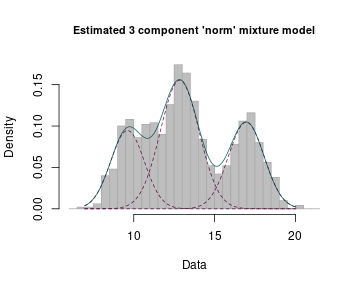At this point, it is worth having a closer look at the thresholds. They each satisfy $$t(j,n) \rightarrow 0$$ as $$n \rightarrow \infty$$, the sole condition the authors require. Now, the consistency proofs for the estimators defined in this Section all rely on the fact that, as $$n \rightarrow \infty$$, $D(\hat{f}_j, \tilde{f}_n) - D(\hat{f}_{j+1}, \tilde{f}_n) \rightarrow d_j > 0, \text{ for } j < p$ and $D(\hat{f}_j, \tilde{f}_n) - D(\hat{f}_{j+1}, \tilde{f}_n) \rightarrow 0, \text{ for } j \geq p,$ where $$p$$ is the true complexity (compare with [@l2, p. 4253, Proof of the Theorem], [@hell, p. 4383, Proof] and [@hellcont, p. 1485, Proof of Theorem 1]. If however $$t(j,n)$$ goes to $$0$$ faster than $$D(\hat{f}_j, \tilde{f}_n) - D(\hat{f}_{j+1}, \tilde{f}_n)$$ for $$j \geq p$$, asymptotically, the decision rule outlined above will always lead to $$j$$ being rejected. Therefore, a second condition should be placed on $$t(j,n)$$, namely choosing it in accordance with $D(\hat{f}_p, \tilde{f}_n) - D(\hat{f}_{p+1}, \tilde{f}_n) = o_p(t(j,n)).$ Both the LIC and the AIC as well as the SBC in the continuous case do not satisfy this condition, yet they are still part of the package for two reasons. First, since they were used in the original papers, they are included for the sake of completeness and reproducibility of original results. Second, consistency is an asymptotic property, and while the aforementioned thresholds do not fulfill it, they still perform well (and not rarely better than consistent thresholds) for smaller sample sizes. In the example above, the number of components is correctly identified under the non-consistent AIC threshold. Nonetheless, the user will get a warning when using one of non-consistent predefined thresholds. The preceding example shows that $$\hat{p}$$ directly depends on the chosen threshold $$t(j, n)$$. While some thresholds can be motivated better than others from a theoretical perspective, the choice will ultimately always remain somewhat arbitrary. It would thus be desirable to have versions of the preceding functions which do not suffer from this drawback. L2.boot.disc, hellinger.boot.disc and hellinger.boot.cont all work similarly to their counterparts, with the exception that the difference in distances is not compared to a predefined threshold but a value generated by a bootstrap procedure. At every iteration (of $$j$$), the procedure sequentially tests $$p = j$$ versus $$p = j+1$$ for $$j = 1,2, \dots$$, using a parametric bootstrap to generate B samples of size $$n$$ from a $$j$$-component mixture given the previously calculated “best” parameter values $$(\hat{\mathbf{w}}^j, \hat{\mathbf{\theta}}^j)$$. For each of the bootstrap samples, again the “best” estimates corresponding to densities with $$j$$ and $$j+1$$ components are calculated, as well as their difference in distances from $$\tilde{f}_n$$. The null hypothesis $$H_0: p = j$$ is rejected and $$j$$ increased by $$1$$ if the original difference $$D(\hat{f}_j, \tilde{f}_n) - D(\hat{f}_{j+1}, \tilde{f}_n)$$ lies outside of the interval $$[ql, qu]$$, specified by the ql and qu empirical quantiles of the bootstrapped differences. Otherwise, $$j$$ is returned as the order estimate $$\hat{p}$$. As an example, consider the poisson rMix object generated in the Section 2. It was a sample from the 3-component poisson mixture corresponding to $$\mathbf{w} = (0.45, 0.45, 0.1)$$ and $$\mathbf{\lambda} = (1, 5, 10)$$. The corresponding datMix object can be generated to use the function hellinger.boot.disc for identifying the number of components as follows: poisList <- vector(mode = "list", length = 1) names(poisList) <- "lambda" poisList$lambda <- c(0, Inf)
MLE.pois <- function(dat) mean(dat)
pois.dM <- RtoDat(poisRMix, theta.bound.list = poisList,
MLE.function = MLE.pois)
set.seed(1)
res <- hellinger.boot.disc(pois.dM, B = 50, ql = 0.025, qu = 0.975)


The results can again be easily visualized.

print(res)
#>
#> Parameter estimation for a 3 component 'pois' mixture model:
#> Function value: 0.003166
#>                     w  lambda
#> Component 1: 0.452735  0.8622
#> Component 2: 0.089649 10.4139
#> Component 3: 0.457616  5.0285
#> Converged in 3 iterations.
#>
#> The estimated order is 3.
plot(res)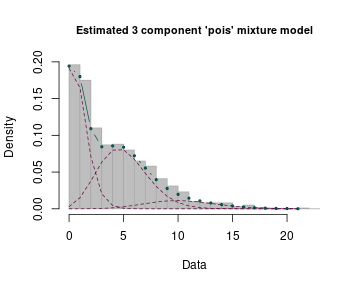## Section 5. Functions using LRTS

As a third option of estimating the mixture complexity, the mixComp package provides an algorithm based on the likelihood ratio test statistic (LRTS), sequentially testing $$p = j$$ versus $$p = j+1$$ for $$j = 1,2, \dots$$, until the algorithm terminates. As noted in Section 1, it is not possible to use the generalized likelihood ratio test in the context of mixture models directly as the standard way of obtaining the asymptotic distribution of the LRTS under the null hypothesis cannot be applied. However, one can get estimates of the test's critical values by employing a bootstrap procedure.

Making use of this approach, the function mix.lrt iteratively increases the assumed order $$j$$ and finds the MLE for both, the density of a mixture with $$j$$ and $$j+1$$ components, giving $$(\hat{\mathbf{w}}^{j}, \hat{\mathbf{\theta}}^{j}) \in W_j \times \Theta_j$$ and $$(\hat{\mathbf{w}}^{j+1}, \hat{\mathbf{\theta}}^{j+1}) \in W_{j+1} \times \Theta_{j+1}$$. It then calculates the corresponding LRTS, defined as $\text{LRTS}= -2\ln\left(\frac{L_0}{L_1}\right) \quad \text{, with}$

$L_0 = L_{\textbf{X}}(\hat{\mathbf{w}}^{j}, \hat{\mathbf{\theta}}^{j}) \quad\quad \text{and} \quad\quad L_1 = L_{\textbf{X}}(\hat{\mathbf{w}}^{j+1}, \hat{\mathbf{\theta}}^{j+1})\text{,}$

$$L_{\textbf{X}}$$ being the likelihood function given the data $${\textbf{X}}$$.

Next, a parametric bootstrap is used to generate B samples of size $$n$$ from a $$j$$-component mixture given the previously calculated MLE $$(\hat{\mathbf{w}}^{j}, \hat{\mathbf{\theta}}^{j})$$. For each of the bootstrap samples, the MLEs corresponding to densities of mixtures with $$j$$ and $$j+1$$ components are calculated, as well as the LRTS. The null hypothesis $$H_0: p = j$$ is rejected and $$j$$ increased by $$1$$ if the LRTS based on the original data vector $$\textbf{X}$$ is larger than the chosen quantile of its bootstrapped counterparts. Otherwise, $$j$$ is returned as the order estimate $$\hat{p}$$. For further details, the reader is referred to [@lrt].

For example, refer back to the waiting variable from the faithful dataset. The mix.lrt function returns a $$2$$-component mixture with reasonable estimates for the component weights and parameters.

set.seed(1)
res <- mix.lrt(faithful.dM, B = 50, quantile = 0.95)
print(res)
plot(res)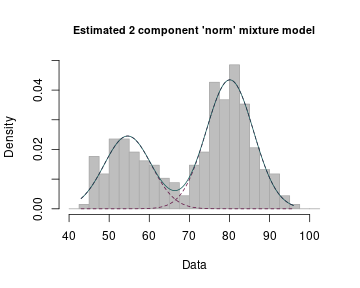## Section 6. Non-standard mixtures

In all preceding examples, the families of component densities $$\{g(x;\theta):\theta \in \Theta \}$$ belonged to one of the “standard” probability distributions included in the stats package, which provides the density/mass function, cumulative distribution function, quantile function and random variate generation for selected distributions. The function names are of the form dxxx, pxxx, qxxx and rxxx respectively. With some additional effort, it is possible to use the mixComp package on “non-standard” distributions – the user merely has to provide functions evaluating the density and generating random numbers for the component distribution. In accordance with R conventions, the user-generated function dxxx has to take x and the distribution parameters as input and returns the value of the density function specifed by the parameters at the point x. Likewise, rxxx requires n and the distribution parameters as input and returns n random numbers based on the distribution specified by the aforementioned parameters.

As an example, consider a sample $$\textbf{X}$$ from a 3-component mixture of normals with means equal to $$10, 11$$ and $$13$$. Assume that the standard deviation of all components is known to be $$0.5$$, yet the number of components and their means are unknown. Then each of the components follows a $$\mathcal{N}(\mu, 0.5)$$ distribution, which shall be called norm0.5. The first step is always that of creating the dxxx and rxxx functions, since they will be called by the mixComp functions.

The following example creates the Mix and rMix objects based on the density of a normal mixture with $$\mathbf{w} = (0.3, 0.4, 0.3)$$, $$\mathbf{\mu} = (10, 11, 13)$$ and $$\mathbf{\sigma} = (0.5, 0.5, 0.5)$$ and plots the obtained mixture density and the corresponding random sample.

dnorm0.5 <- function(x, mean){
dnorm(x, mean = mean,  sd = 0.5)
}
rnorm0.5 <- function(n, mean){
rnorm(n, mean = mean,  sd = 0.5)
}
## create objects Mix and rMix:
set.seed(1)
norm0.5Mix <- Mix("norm0.5", w = c(0.3, 0.4, 0.3), mean = c(10, 11, 13))
norm0.5RMix <- rMix(1000, obj = norm0.5Mix)
## plot the results:
plot(norm0.5Mix)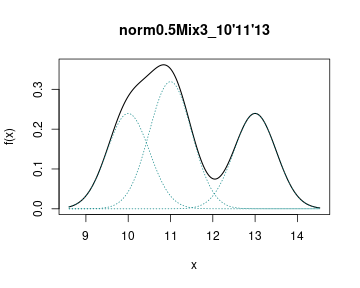plot(norm0.5RMix)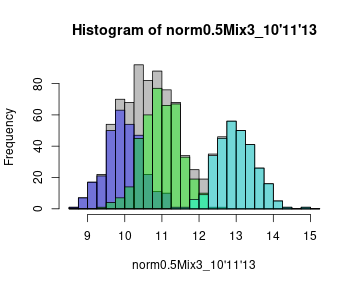Below we will estimate of the mixture density using mix.lrt given a sample from the 3-component normal mixture with $$\mathbf{w} = (0.3, 0.4, 0.3)$$, $$\mathbf{\mu} = (10, 11, 13)$$, $$\mathbf{\sigma} = (0.5, 0.5, 0.5)$$.

We start by creating all necessary inputs:

norm0.5.list <- vector(mode = "list", length = 1)
names(norm0.5.list) <- c("mean")
norm0.5.list\$mean <- c(-Inf, Inf)

MLE.norm0.5 <- function(dat) mean(dat)

norm0.5.dM <- RtoDat(norm0.5RMix, theta.bound.list = norm0.5.list,
MLE.function = MLE.norm0.5)


Finally, the mixComp procedures can be used on the datMix object as usual.

set.seed(1)
res <- mix.lrt(norm0.5.dM, B = 50, quantile = 0.95)


The results can be printed and plotted using print and plot functions.

print(res)
#>
#> Parameter estimation for a 3 component 'norm0.5' mixture model:
#> Function value: 1543.7935
#>                    w   mean
#> Component 1: 0.32686 10.010
#> Component 2: 0.29273 12.983
#> Component 3: 0.38041 11.034
#> Converged in 3 iterations.
#>
#> The estimated order is 3.
plot(res)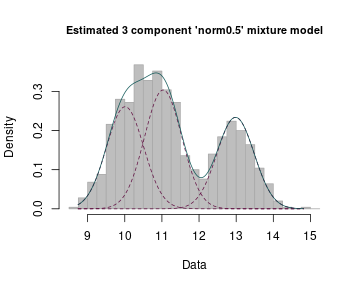## Section 7. Summary and discussion

In this paper we presented the R package mixComp, a collection of routines developed to estimate a mixture's complexity from a data sample $$\textbf{X}$$. Moreover, it provides the possibility of generating artificial data from specified mixtures as well as proper visualization tools for the complexity estimation result, plotting either the successive determinant values or the final fitted mixture. If estimates of the component weights and parameters are sought, $$\hat{p}$$ can be passed to one of the many R packages specialized in their calculation, or one of the mixComp functions returning weight and parameter estimates can be used. However, it should be noted that the theory on which this package is based concentrates on showing consistency for $$\hat{p}$$ – other estimates obtained in the process are merely by-products. A primary goal of the package was to make it extendible, meaning that it can be utilized on mixtures of less well-known distributions, as long as sufficient information on the density and random variate generation is provided. We hope that this package will be useful for practitioners in the many areas where mixture models are applicable.

## Computational details

All computations and graphics in this paper have been done using R version 4.0.0 with the packages boot 1.3-24, cluster 2.1.0, expm 0.999-4, matrixcalc 1.0-3, Rsolnp 1.16 and kdensity 1.0.1. R itself and all packages used are available from the Comprehensive R Archive Network (CRAN) at \url{https://CRAN.R-project.org/}.

## Appendix

### Distance and non-parametric estimator definitions

Let $$\textbf{X} = \{X_1, \dots, X_n\}$$ be an i.i.d. sample of size $$n$$ from some continuous distribution with unknown density $$f$$. Its kernel densty estimator is defined as $\tilde{f}_n(x):= \frac{1}{nc_n}\sum_{i=1}^n K\left( \frac{x-X_i}{c_n} \right),$ with kernel $$K$$ and bandwidth $$c_n$$.

$\tilde{f}_n(x) := \frac{1}{n}\sum_{i=1}^n \sum_{j=1}^k \frac{a_j(X_i)}{c_{n, j}} K\left( \frac{x-X_i}{c_{n, j}} \right),$

with kernel $$K$$, bandwidth $$c_{n, j}$$ and weights (positive and summing to $$1$$) $$a_j(X_i)$$.

Let $$\textbf{X} = \{X_1, \dots, X_n\}$$ be an i.i.d. sample of size $$n$$ from some discrete distribution with unknown probability mass function $$f$$. Its empirical probability mass function is defined as $\tilde{f}_n(x) := \frac{1}{n}\sum_{i=1}^n \mathbf{1}_{\{X_i = x\}}.$

Let $$g, f$$ be two probability mass functions defined on $$\mathcal{X}$$. The squared $$L_2$$ distance between $$g$$ and $$f$$ is given by $L_2^2(g,f) := \sum_{\mathcal{X}} (g(x)-f(x))^2.$

Let $$g, f$$ be two density or probability mass functions defined on $$\mathcal{X}$$. The squared Hellinger distance between $$g$$ and $$f$$ is given by $H^2(g,f) := \sum_{\mathcal{X}} \left( \sqrt{g(x)}-\sqrt{f(x)} \right)^2$ in the discrete case and by $H^2(g,f) := \int_{\mathcal{X}} \left( \sqrt{g(x)}-\sqrt{f(x)} \right)^2 dx$ in the continuous case.

\pagebreak